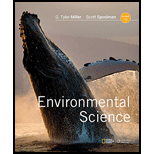Chapter 2, Problem 3CREnvironmental Science (MindTap Cou...

16th Edition
G. Tyler Miller + 1 other
ISBN: 9781337569613

Solutions

Chapter
SectionEnvironmental Science (MindTap Cou...

16th Edition
G. Tyler Miller + 1 other
ISBN: 9781337569613
Textbook Problem

Define scientific law, or law of nature, and give an example. Explain why scientific theories and laws are the most important and most certain results of science and why people often use the term theory incorrectly.

To determine

The definition of scientific law and its example. The reason that scientific theories and laws are important concepts in scientific studies. The reason for the incorrect use of the term “theory.”

Explanation

A scientific theory is an important concept in scientific studies. It is also known as the ‘law of nature”. The information about natural processes is tested and validated by the help of hypothesis testing and experimentations. The validated information that is widely accepted by a large number of people is termed as a scientific theory. A theory arises from a hypothesis. A proposed hypothesis that holds true and does not change due to minute environmental changes becomes a scientific theory.

An example of a scientific theory is the “law of gravity.”  The scientists conducted various experiments to validate a hypothesis that states “every falling object reaches the earth due to a gravitational force.” It was observed that the hypothesis holds true for all the objects. As a result, “law of gravity” was widely accepted and it became a scientific theory.

A scientific theory is the most important part of science as it provides information about the existence of a true phenomenon...

Still sussing out bartleby?

Check out a sample textbook solution.

See a sample solution

The Solution to Your Study Problems

Bartleby provides explanations to thousands of textbook problems written by our experts, many with advanced degrees!

Get Started